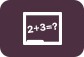# Free Basic Math WorksheetsFree Basic Math Worksheets, Printables, and Activities.

All of our Basic Math Worksheets are available for free downloading and printing for students, teachers, and homeschool. They cover a variety of basic math topics and skills including addition, subtraction, measurement conversions, basic counting, fractions and more.

This free worksheet contains problems where students must write numbers in expanded form and index notation. Other problems include using leading number to estimate operations, write statements using...
Worksheet (Pre-Algebra)
Problems: 6
In this free math worksheet, students must divide and simplify fractions and mixed numbers. All problems contain only numbers (no variables).
Worksheet (Pre-Algebra)
Problems: 15
This free basic math worksheet contains problems where students must convert between different units of time such as millennium to years, hours to days, seconds to minutes, and so on. Other...
Worksheet (Basic Math)
Problems: 20
In this free basic math worksheet, students must convert between measurements such as km to m, kg to tons, minutes to hours, days to hours, and so on. There are also problems on scientific notation.
Worksheet (Basic Math)
Problems: 19
In this free basic math worksheet (1st grade level), students must complete problems by counting by 2 and filling in missing blanks. Problems contain blocks of 10 numbers each starting with smaller...
Worksheet (Basic Math)
Problems: 13
In this free 1st grade level basic math worksheet, students count by 1 in blocks of 10 by filling in blanks. By the end of the worksheet students will count up to 50.
Worksheet (Basic Math)